# SAS/STAT Examples

## Bayesian Hierarchical Modeling for Meta-Analysis

Contents | SAS Program | PDF

## Overview

Meta-analysis is an important technique that combines information from different studies. When you have no prior information for thinking any particular study is different from another, you can treat Bayesian meta-analysis as a hierarchical model. This example illustrates two different applications of hierarchical modeling to a meta-analysis in medicine. The first application uses a normal approximation to the likelihood, and the second application uses the exact binomial likelihood.

# Analysis

Consider the data from Yusuf et al. (1985), which summarize mortality after myocardial infarction from 22 studies. For each study, the data are in the form oftables that consist of patients who are randomly assigned to receive beta-blockers or placebo. For study, suppose that trtis the number of deaths out of trtNpatients in the treatment group and ctrlis the number of deaths out of ctrlNpatients in the control group.

The following statements read the data into SAS and create the Multistudies data set:

```data multistudies;
input studyid ctrl ctrlN trt trtN;
datalines;
1 3 39 3 38
2 14 116 7 114
3 11 93 5 69
4 127 1520 102 1533
5 27 365 28 355
6 6 52 4 59
7 152 939 98 945
8 48 471 60 632
9 37 282 25 278
10 188 1921 138 1916
11 52 583 64 873
12 47 266 45 263
13 16 293 9 291
14 45 883 57 858
15 31 147 25 154
16 38 213 33 207
17 12 122 28 251
18 6 154 8 151
19 3 134 6 174
20 40 218 32 209
21 43 364 27 391
22 39 674 22 680
;
```

Suppose you want use a Bayesian random-effects model to estimate both the study-specific treatment effect and the pooled treatment effect. A Bayesian random-effects model assumes that there is no prior information for thinking that one study is different from the other. This assumption is also known as exchangeability.

## Hierarchical Model Using Normal Approximation to the Likelihood

Normal approximation to binomial likelihood is a classical method that is commonly used in meta-analysis. Letbe the odds ratio for theth study: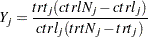You can estimate the treatment effect,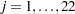, through the means of an approximate normal distribution,whereis the study-specific effect andis the known variance of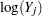. For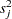, because all studies have large sample sizes (more than 50 persons in each group in nearly all of the studies), you can use the approximate sampling variance of:If the odds ratios are exchangeable between the studies, then the treatment effect in each trial can be considered to be a random quantity drawn from some population distribution. In a Bayesian framework, this means that you can place a common prior distribution on the exchangeable random-effects parameters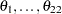,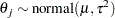whereis the population average of the treatment effect across all studies and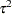is the between-study variation. Suppose the following noninformative priors are placed on the hyperparametersand: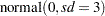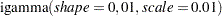The following DATA step creates the response variable,, and its approximate sampling variance,for.

```data multistudies;
set multistudies;
logy=log(trt/(trtN-trt)/(ctrl/(ctrlN-ctrl)));
sigma2=1/trt+1/(trtN-trt)+1/ctrl+1/(ctrlN-ctrl);
run;
```

The following MCMC procedure statements fit the described random-effects model:

```proc mcmc data=multistudies outpost=nlout seed=276 nmc=50000 thin=5
monitor=(OR Pooled);
array OR;
parms  mu tau2;
prior mu ~ normal(0, sd=3);
prior tau2~ igamma(0.01,s=0.01);
random theta ~n(mu, var=tau2) subject=studyid;
OR[studyid]=exp(theta);
Pooled=exp(mu);
model logy ~ n(theta, var=sigma2);
run;
```

The PROC MCMC statement specifies the input data set as Multistudies and the output data set as Nlout, sets a seed for the random number generator, and requests a large simulation size with thinning. The MONITOR= option in the PROC MCMC statement outputs the analyses or saves the posterior samples for the specified variables. The ARRAY statement allocates an array variable OR of size 22, which is the number of random-effects parameters. The PARMS statement declaresandas model parameters, and the PRIOR statements specify their prior distributions.

The RANDOM statement declaresas random-effects parameters and specifies the normal prior distribution with hyperparametersand. The SUBJECT= option in the RANDOM statement specifies the group index as the data set variable studyid. The RANDOM statement generates 22 random-effects parameters, one for each unique value in the studyid variable. To monitor the function of 22 random-effects parameters, you must allocate an array and store the transformation of every random-effects parameter. The next programming statement, OR[studyid]=exp(theta) , does precisely this. As the procedure steps through the data set, it calculates the exponential of eachparameter and stores the result in theth element of the OR array according to the studyid variable value (indexing). For this statement to work, the subject index variable studyid must have an integer value (1, 2, 3, and so on). The symbol Pooled calculates the exponential of the hyperparameter, which is the pooled treatment effect. The MODEL statement specifies the normal likelihood for the log odds ratios.

Figure 1 reports posterior summary statististics for each of the 22 odds ratios and for the Pooled estimate. The odds ratios provide the multiplicative change in the odds of mortality in the treatment group with respect to the control group. Some variation exists among the treatment effects. The pooled estimate of the odds ratio is 0.7849, indicating that mortality is less likely in the treatment groups.

Figure 1 Posterior Summaries for the Normal Approximation Model
The MCMC Procedure

Posterior Summaries
Parameter N Mean Standard
Deviation
Percentiles
25% 50% 75%
OR1 10000 0.8038 0.1471 0.7112 0.7879 0.8749
OR2 10000 0.7545 0.1253 0.6764 0.7523 0.8285
OR3 10000 0.7752 0.1313 0.6913 0.7663 0.8494
OR4 10000 0.7863 0.0852 0.7284 0.7809 0.8387
OR5 10000 0.8563 0.1366 0.7629 0.8370 0.9248
OR6 10000 0.7791 0.1370 0.6924 0.7697 0.8529
OR7 10000 0.6829 0.0776 0.6312 0.6835 0.7349
OR8 10000 0.8381 0.1116 0.7628 0.8269 0.9007
OR9 10000 0.7544 0.1095 0.6829 0.7499 0.8216
OR10 10000 0.7449 0.0705 0.6964 0.7423 0.7896
OR11 10000 0.7978 0.1007 0.7294 0.7910 0.8572
OR12 10000 0.8430 0.1203 0.7609 0.8293 0.9073
OR13 10000 0.7580 0.1211 0.6797 0.7536 0.8286
OR14 10000 0.9573 0.1570 0.8469 0.9297 1.0455
OR15 10000 0.7793 0.1166 0.7023 0.7727 0.8458
OR16 10000 0.8151 0.1202 0.7334 0.8052 0.8803
OR17 10000 0.8454 0.1429 0.7516 0.8250 0.9157
OR18 10000 0.8322 0.1525 0.7349 0.8122 0.9061
OR19 10000 0.8232 0.1511 0.7284 0.8036 0.8941
OR20 10000 0.7964 0.1168 0.7195 0.7847 0.8621
OR21 10000 0.7202 0.1067 0.6484 0.7191 0.7879
OR22 10000 0.7218 0.1055 0.6541 0.7222 0.7866
Pooled 10000 0.7849 0.0525 0.7498 0.7826 0.8166

You can useCATER autocall macro to create a caterpillar plot of the odds ratios. The following statement takes the output from the Nlout data set and generates a caterpillar plot of all study-specific odds ratios and the pooled odds ratio:

```%CATER(data=nlout, var=OR: Pooled);
```

Figure 2 shows the posterior means and the 95equal-tail credible intervals of odds ratios from the 22 studies and for the pooled odds ratio. It looks like the treatment is most effective for Study 7 and least effective for Study 14. This result illustrates another advantage of the random-effects models: a fixed-effects model focuses on the coefficient effects after averaging study differences, whereas a random-effects model can help identify studies that are very different from others, such as ones with very high or low treatment effects.

Figure 2 Caterpillar Plot of the Odds Ratios for the Normal Approximation Model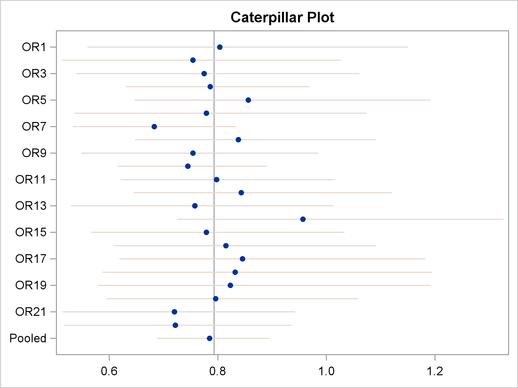## Hierarchical Model Using Binomial Likelihood

The standard normal approximation method might not be appropriate because the approximation becomes less precise in the extreme probabilities (for example, 0,1). In the Multistudies data set, the mortality rates in the thirteenth study and nineteenth study are closest to 0 (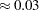). Therefore, an alternative approach is to use the exact likelihood approach as opposed the normal approximation.

Emulating the binomial model as outlined in Spiegelhalter, Abrams, and Myles (2004), you can use the following model, where the treatment and control groups have their own binomial likelihood functions, with death probabilities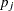and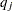in the treatment group and control group, respectively: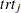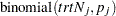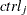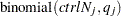Let the log odds for the control group beand let the log odds for the treatment group beas follows:Thenis the log odds ratio: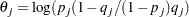If treatment group risks are exchangeable, then you can assume thatare random-effects parameters that are drawn from some common prior distribution: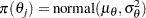If the control group risks are also exchangeable, then you can consideras other random-effects parameters with the following common prior: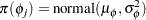Suppose the following priors are placed on the model parameters: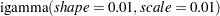The following PROC MCMC statements fit the described model:

```proc mcmc data=multistudies outpost=blout seed=126 nmc=50000 thin=5
monitor=(OR Pooled);
array OR;
parms  mu_theta mu_phi s_theta s_phi;
prior mu:~ normal(0,sd=3);
prior s:~ igamma(0.01,s=0.01);
random theta ~n(mu_theta, var=s_theta) subject=studyid;
random phi ~n(mu_phi, var=s_phi) subject=studyid;
p = logistic(theta + phi);
q = logistic(phi);
model trt ~ binomial(trtN, p);
model ctrl ~ binomial(ctrlN, q);
OR[studyid]=exp(theta);
Pooled=exp(mu_theta);
run;

```

The PROC MCMC statement specifies the input data set as Multistudies and the output data set as Blout, sets a seed for the random number generator, and requests a large simulation size with thinning. The MONITOR= option in the PROC MCMC statement saves the posterior samples for the specified variables. The ARRAY statement allocates an array variable OR of size 22. The PARMS statement declares, and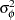as model parameters, and the PRIOR statements specify their prior distributions. The RANDOM statements declareandas random-effects parameters and specify a normal prior distribution for them. The SUBJECT= option in both RANDOM statements indicates the group index as data set variable studyid. The LOGISTIC functions perform logistic transformation from the log odds of the treatment and control groups to the corresponding binomial mortality probabilities. The MODEL statements specify the binomial likelihood for the treatment and the control groups. The programming statement in the next line, OR[studyid]=exp(theta) , calculates the exponential of each ‘theta’ effect and stores the result in the OR array according to the studyid variable value (indexing). The symbol Pooled calculates the exponential of the hyperparameter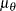, which is the pooled treatment effect.

Figure 3 reports posterior summary statististics for each of the 22 odds ratios.

Figure 3 Posterior Summaries for the Binomial Model
The MCMC Procedure

Posterior Summaries
Parameter N Mean Standard
Deviation
Percentiles
25% 50% 75%
OR1 10000 0.7960 0.1410 0.7066 0.7867 0.8668
OR2 10000 0.7477 0.1221 0.6698 0.7428 0.8202
OR3 10000 0.7734 0.1308 0.6913 0.7639 0.8460
OR4 10000 0.7801 0.0817 0.7230 0.7758 0.8308
OR5 10000 0.8461 0.1322 0.7573 0.8274 0.9163
OR6 10000 0.7771 0.1345 0.6924 0.7656 0.8515
OR7 10000 0.6868 0.0762 0.6346 0.6859 0.7376
OR8 10000 0.8377 0.1113 0.7626 0.8286 0.9012
OR9 10000 0.7583 0.1104 0.6854 0.7533 0.8250
OR10 10000 0.7402 0.0701 0.6907 0.7386 0.7864
OR11 10000 0.7947 0.1016 0.7270 0.7854 0.8570
OR12 10000 0.8577 0.1288 0.7692 0.8414 0.9259
OR13 10000 0.7365 0.1201 0.6592 0.7334 0.8094
OR14 10000 0.9382 0.1443 0.8361 0.9168 1.0183
OR15 10000 0.7954 0.1174 0.7185 0.7849 0.8625
OR16 10000 0.8290 0.1265 0.7470 0.8138 0.8961
OR17 10000 0.8502 0.1424 0.7578 0.8290 0.9196
OR18 10000 0.8082 0.1391 0.7171 0.7908 0.8819
OR19 10000 0.7840 0.1338 0.6993 0.7748 0.8561
OR20 10000 0.8078 0.1185 0.7279 0.7948 0.8771
OR21 10000 0.7190 0.1049 0.6503 0.7182 0.7879
OR22 10000 0.7064 0.1077 0.6348 0.7055 0.7753
Pooled 10000 0.7810 0.0529 0.7446 0.7795 0.8151

You can useCATER autocall macro to create a caterpillar plot of the odds ratios. The following statement takes the output from the Nlout data set and generates a caterpillar plot of all study-specific treatment effects and the overall treatment effect:

```%CATER(data=blout, var=OR: Pooled);
```

Figure 4 showscredible intervals of odds ratios from the 22 studies. Comparing Figure 2 and Figure 4, you can see that the posterior means and the credible intervals that are obtained by using the approximate normal likelihood and the binomial likelihood are very similar.

Figure 4 Caterpillar Plot of the Odds Ratios for the Binomial Model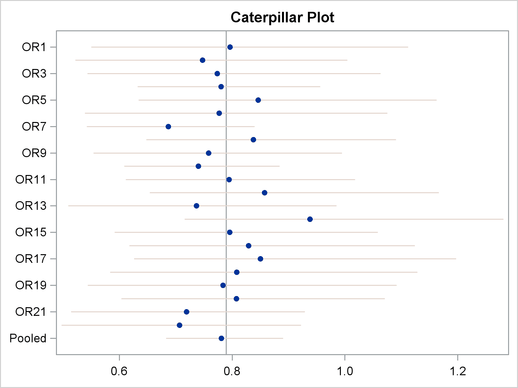Figure 5 compares the kernel density plots of the odds ratios that are produced by using the approximate normal likelihood and the exact binomial likelihood. The first plot shows the comparison for the thirteenth study, and the second plot shows the comparison for the nineteenth study. Even though the observed mortality rates () are close to 0 for both studies, the kernel density plots that are produced by using the approximate normal likelihood and the exact binomial likelihood are very similar. Hence, this example shows very little sensitivity to the likelihood assumption. In general, this can be explained by the large sample sizes of the studies included in the meta-analysis.

Figure 5 Kernel Density Comparison of Odds Ratios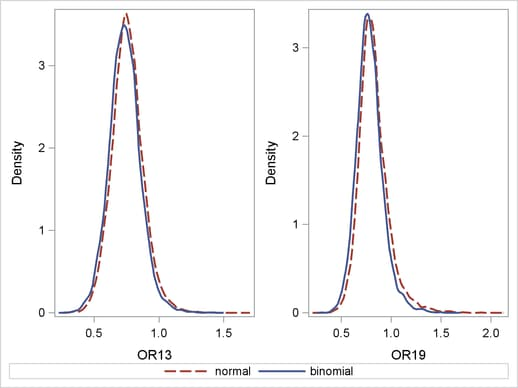# References

Spiegelhalter, D. J., Abrams, K. R., and Myles, J. P. (2004), Bayesian Approaches to Clinical Trials and Health-Care Evaluation, Chichester, England: John Wiley & Sons.

Yusuf, S., Petro, R., Lewis, J., Collins, R., and Sleight, P. (1985), “Beta Blockade During and After Myocardial Infarction: An Overview of Randomized Trials,” Progress in Cardiovascular Diseases, 27, 335–371.